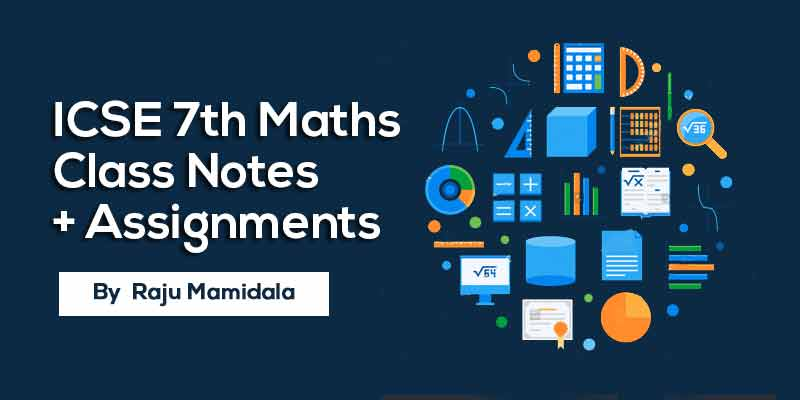## by Raju Mamidala

51 Users Enrolled
Class 7 , Maths
Board: ICSE , Language: English

Mode- Online
Contains- 38 PDFS
Access Duration- Till 30 Apr 2019

## WHAT WILL I LEARN?

We aim to prepare Class 7 ICSE students with a complete study material so that the students can score better in the school exam.

Simple and easy to understand.

Understand the level of IIT Foundation concepts by these quick revision notes.

Student can test themself with assignments which are provided here.

## Course Description

ICSE Class 7 is an important stage where students need to concentrate on this subject as it is considered to be the groundwork of higher classes. To become clear with your aspiration and knowledge, you need to begin your preparation from this level. so here you can get complete notes and assignments for preparation along with solutions/Answers.

This study material will help you to better understand the topics and  these notes, students will be able to make quick revisions of topics and score good marks in there exams.

## Curriculum

ALGEBRAIC EXPRESSIONS

AVERAGE, Time AND Work , time and distance

DECIMALS

• DECIMALS - Class Notes
• DECIMALS - Work Assignment

FACTORS _ MULTIPLES

• FACTORS _ MULTIPLES - Class Notes
• FACTORS _ MULTIPLES - Work Assignment

FRACTIONS

• FRACTIONS - Class Notes
• FRACTIONS - Work Assignment

FUNDAMENTAL GEOMETRICAL concepts, line angles and circle

• FUNDAMENTAL GEOMETRICAL concepts line angles and circle - Class Notes
• FUNDAMENTAL GEOMETRICAL concepts line angles and circle - Work Assignment

LINEAR EQUATIONS, inequations and graphs

• LINEAR EQUATIONS, inequations and graphs - Class Notes
• LINEAR EQUATIONS, inequations and graphs - Work Assignment

NUMBER SYSTEM

• NUMBER SYSTEM - Class Notes
• NUMBER SYSTEM - Work Assignment

PERCENTAGE, PROFIT, LOSS and discount , simple interest

• PERCENTAGE, PROFIT, LOSS and discount , simple interest - Class Notes
• PERCENTAGE, PROFIT, LOSS and discount , simple interest - Work Assignment

PERIMETER AND AREA, volume and surface area of solids

• PERIMETER AND AREA, volume and surface area of solids - Class Notes
• PERIMETER AND AREA, volume and surface area of solids - Work Assignment

• Polygons and quadrilaterals - Class Notes
• Polygons and quadrilaterals - Work Assignment

POWER AND ROOTS and exponents

• POWER AND ROOTS and exponents - Class Notes
• POWER AND ROOTS and exponents - Work Assignment

RATIO, PROPORTION and unitary method

• RATIO, PROPORTION and unitary method - Class Notes
• RATIO, PROPORTION and unitary method - Work Assignment

RELATIONS AND mapping

• RELATIONS AND mapping - Class Notes
• RELATIONS AND mapping - Work Assignment

SET AND VENN diagram

• SET AND VENN diagram - Class Notes
• SET AND VENN diagram - Work Assignment

SPECIAL PRODUCTS AS identities formula and factorization

• SPECIAL PRODUCTS AS identities formula and factorization - Class Notes
• SPECIAL PRODUCTS AS identities formula and factorization - Work Assignment

STATISTICS

• STATISTICS - Class Notes
• STATISTICS - Work Assignment

SYMMETRY, REFLECTION and rotation

• SYMMETRY, REFLECTION and rotation - Class Notes
• SYMMETRY, REFLECTION and rotation - Work Assignment

TRIANGLES, CONSTRUCTION OF triangles and congruence of triangles

• TRIANGLES, CONSTRUCTION OF triangles and congruency of triangles - Class Notes
• TRIANGLES, CONSTRUCTION OF triangles and congruency of triangles - Work Assignment

## About Raju Mamidala### Raju Mamidala

Mathematics | Class 6th to Competitive exams | 15+ Years Experience | Working in Geethanjali International School in Hyderabad from past 5 years. He Created an Educational Channel for improvement in various aspects for students in IIT Foundation.

#### If you have any doubts about how to get and access this course,watch this video.## 500 299

Includes 18 % GST

Mode- Online

Contains- 38 PDFS

Access Duration- Till 30 Apr 2019